Solution of Gravitation (Page No - 126) - Physics by Lakhmir Singh, Class 9

# Solution of Gravitation (Page No - 126) - Physics by Lakhmir Singh, Class 9 - Extra Documents & Tests for Class 9

Question 59:
(a) What are fluids ? Name two common fluids.
(b) State Archimedes’ principle.
(c) When does an object float or sink when placed on the surface of a liquid ?

Solution :
(a) Those substances which can flow easily are called fluids. All the liquid and gases are fluids, like water, air etc.
(b) Archimedes’ Principle :
When an object is wholly (or partially) immersed in a liquid, it experiences a buoyant force (or upthrust) which is equal to the weight of liquid displaced by the object.
Buoyant force on an object = weight of liquid displaced by that object
(c) If the buoyant force exerted by the liquid is less than the weight of the object, the object will sink in the liquid. If the buoyant force exerted by the liquid is equal to or greater than the weight of the object, the object will float in the liquid.

Question 60:
(a) How does a boat float in water ?
(b) A piece of steel has a volume of 12 cm3, and a mass of 96 g. What is its density :
(i) in g/cm3 ?
(ii) in kg/m3 ?

Solution :
(a) A floating boat displaces water equal to its own weight. This displaced water exerts buoyant force to balance the weight of boat and keep it afloat.
(b) (i) Mass = 96 g
Volume = 12cm3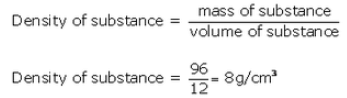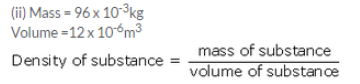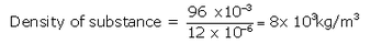Question 61:
An elephant weighing 40,000 N stands on one foot of area 1000 cm2 whereas a girl weighing 400 N is standing on one ‘stiletto’ heel of area 1 cm2.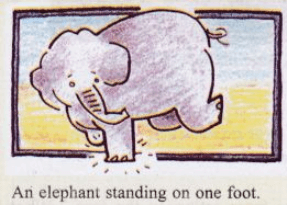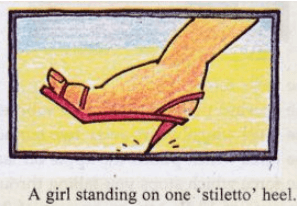(a) Which of the two, elephant or girl, exerts a larger force on the ground and by how much ?
(b) What pressure is exerted on the ground by the elephant standing on one foot ?
(c) What pressure is exerted on the ground by the girl standing on one heel ?
(d) Which of the two exerts larger pressure on the ground : elephant or girl ?
(e) What is the ratio of pressure exerted by the girl to the pressure exerted by the elephant ?
Solution :
Weight of elephant=40000N
Area of one foot =1000 cm2= 1000 x 10-4m2
Weight of girl=400N
Area of heel of girl =1 cm2=1 x 10-4m2
(a) Elephant has a larger weight of 40000N, therefore, elephant exerts a larger force on the ground. Elephant exerts a larger force on the ground by 40000N – 400 N=39600N.
(b)Weight of elephant = 40000N
Area of one foot =1000cm2= 1000 x 10-4m2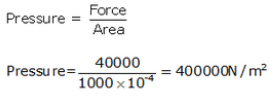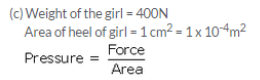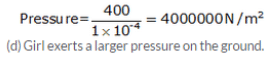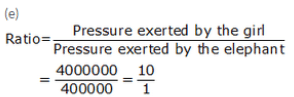The pressure exerted by girl is 10 times greater than the exerted by the elephant.

The document Solution of Gravitation (Page No - 126) - Physics by Lakhmir Singh, Class 9 | Extra Documents & Tests for Class 9 is a part of the Class 9 Course Extra Documents & Tests for Class 9.
All you need of Class 9 at this link: Class 9

## Extra Documents & Tests for Class 9

1 videos|228 docs|21 tests

## FAQs on Solution of Gravitation (Page No - 126) - Physics by Lakhmir Singh, Class 9 - Extra Documents & Tests for Class 9

 1. What is the formula for gravitational force between two objects?Ans. The formula for gravitational force between two objects is given by Newton's law of universal gravitation, which states that the force is directly proportional to the product of the masses of the objects and inversely proportional to the square of the distance between their centers. Mathematically, it can be expressed as F = G * (m1 * m2) / (r^2), where F is the gravitational force, G is the gravitational constant, m1 and m2 are the masses of the objects, and r is the distance between their centers.
 2. How does the mass of an object affect the gravitational force acting on it?Ans. The mass of an object directly affects the gravitational force acting on it. According to Newton's law of universal gravitation, the gravitational force is directly proportional to the product of the masses of the objects. This means that as the mass of an object increases, the gravitational force acting on it also increases. Therefore, a larger mass will experience a stronger gravitational force compared to a smaller mass.
 3. What is the difference between mass and weight?Ans. Mass and weight are two different physical quantities. Mass is the amount of matter present in an object and is a scalar quantity. It is constant and does not change with location. On the other hand, weight is the force with which an object is attracted towards the center of the Earth due to gravity. It is a vector quantity and can vary depending on the location. Weight is directly proportional to the mass of the object and the acceleration due to gravity.
 4. How does the distance between two objects affect the gravitational force between them?Ans. According to Newton's law of universal gravitation, the gravitational force between two objects is inversely proportional to the square of the distance between their centers. This means that as the distance between two objects increases, the gravitational force between them decreases. Similarly, if the distance decreases, the gravitational force increases. Thus, the distance between two objects has a significant impact on the magnitude of the gravitational force between them.
 5. What is the value of the gravitational constant?Ans. The value of the gravitational constant, denoted by G, is approximately 6.67430 × 10^-11 Nm^2/kg^2. It is a fundamental constant in physics and plays a crucial role in determining the strength of the gravitational force between two objects. The value of G is constant and does not change. It is used in the formula for gravitational force to calculate the magnitude of the force between two objects based on their masses and the distance between them.

## Extra Documents & Tests for Class 9

1 videos|228 docs|21 testsExplore Courses for Class 9 examSignup to see your scores go up within 7 days! Learn & Practice with 1000+ FREE Notes, Videos & Tests.
10M+ students study on EduRev
Track your progress, build streaks, highlight & save important lessons and more!
Related Searches

,

,

,

,

,

,

,

,

,

,

,

,

,

,

,

,

,

,

,

,

,

,

,

,

;